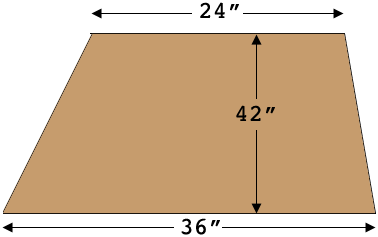SEARCH HOMEMath Central Quandaries & QueriesQuestion from Peter: I'm building a dry stack stone wall which is 36” wide at the base, 42” tall and 24” wide at the top. What would be the volume for a 36” long segment ? Thanks, PeterHi Peter,

The shape of the cross section of your wall is a trapezoid.The area of a trapezoid is the average of the lengths of the parallel sides, times the distance between the parallel sides In your case that's

$\frac{36 + 24}{2}\times 42 \mbox{ square inches.}$

To calculate the volume of a section of the wall in cubic inches multiply the cross section area by the length in inches, in your case 36 inches.

Cheers,
PennyMath Central is supported by the University of Regina and The Pacific Institute for the Mathematical Sciences.